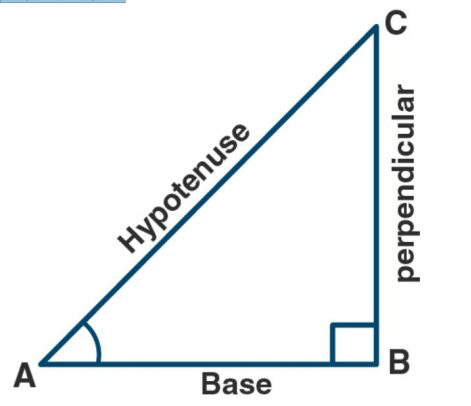# ML Aggarwal Solutions Class 9 Mathematics Solutions for Trigonometric Ratios Exercise 17 in Chapter 17 - Trigonometric Ratios

Given A is an acute angle and 13 sin A 5, evaluate: (5 sin A – 2 cos A)/ tan A

The ratio of lengths of sides opposing the angle and the hypotenuse defines the sine of an angle. It is symbolised by the letter sinθ

The ratio of lengths of neighbouring sides to the angle and the hypotenuse defines the cosine of an angle. It is denoted by the symbol cosθ

The ratio of the length of the sides opposite the angle and the side adjacent to the angle defines the tangent of an angle. Tanθ is the symbol for it.

Let triangle ABC be a right-angled triangle at B and A is an acute angle

Given that 13 sin A = 5

Sin A = 5/13

AB/Ac = 5/13Let AB = 5x

AC = 13 x

In right-angled triangle ABC,

Using Pythagoras theorem,

We get

\begin{array}{l} A C^{2}=A B^{2}+B C^{2} \\ B C^{2}=A C^{2}-B C^{2} \\ B C^{2}=(13 x)^{2}-(5 x)^{2} \\ B C^{2}=169 x^{2}-25 x^{2} \\ B C^{2}=144 x^{2} \\ B C=12 x \\ \sin A=5 / 13 \end{array}

Cos A = base/ hypotenuse

= BC/AC

= 12x/ 13x

= 12/13

Tan A = perpendicular/ base

= AB/BC

= 5x/ 12x

= 5/ 12

Now,

(5 sin A – 2 cos A)/ tan A = [(5) (5/13) – (2) (12/13)]/ (5/12)

= (1/13)/ (5/12)

= 12/65

Hence (5 sin A – 2 cos A)/ tan A = 12/65

Related Questions
Exercises

Lido

Courses

Teachers

Book a Demo with us

Syllabus

Maths
CBSE
Maths
ICSE
Science
CBSE

Science
ICSE
English
CBSE
English
ICSE
Coding

Terms & Policies

Selina Question Bank

Maths
Physics
Biology

Allied Question Bank

Chemistry
Connect with us on social media!